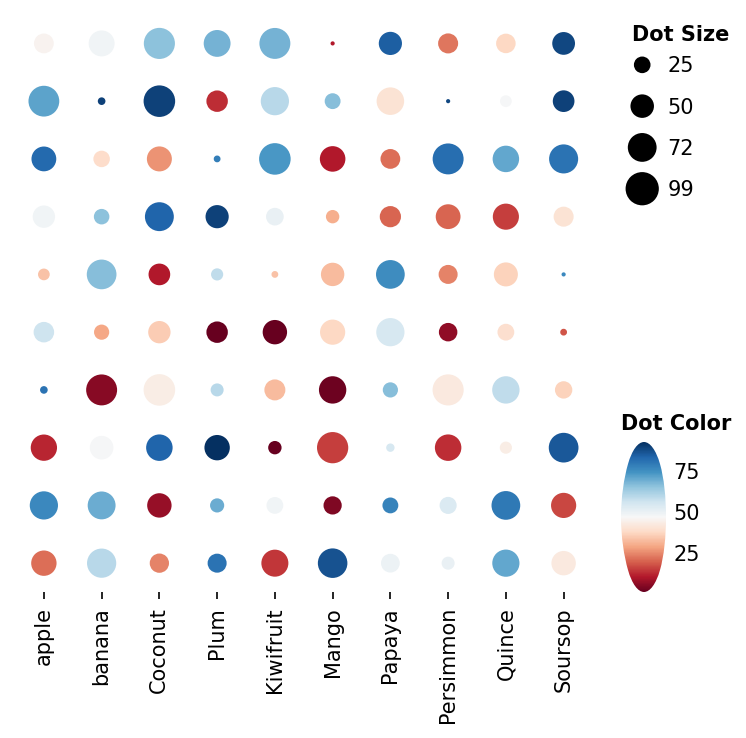# Dot heatmap Example#

Here shows how to draw dot heatmap

```import numpy as np
import milkviz as mv
```

## First let’s create some random data#

```np.random.seed(0)
shape = (10, 10)
colors = np.random.randint(1, 100, shape)
sizes = np.random.randint(1, 100, shape)
matrix = np.random.randint(1, 100, shape)
labels = ["apple", "banana", "Coconut", "Plum", "Kiwifruit",
"Mango", "Papaya", "Persimmon", "Quince", "Soursop"]
```

## Create the dot heatmap#

```mv.dot_heatmap(sizes, colors, xticklabels=labels,
dot_size_legend_kw={"title": "Dot Size"},
dot_hue_cbar_kw={"title": "Dot Color"},
)
```Out:

```<milkviz._dot_matrix.DotHeatmap object at 0x7fc33db9eca0>
```

Total running time of the script: ( 0 minutes 0.697 seconds)

Gallery generated by Sphinx-Gallery